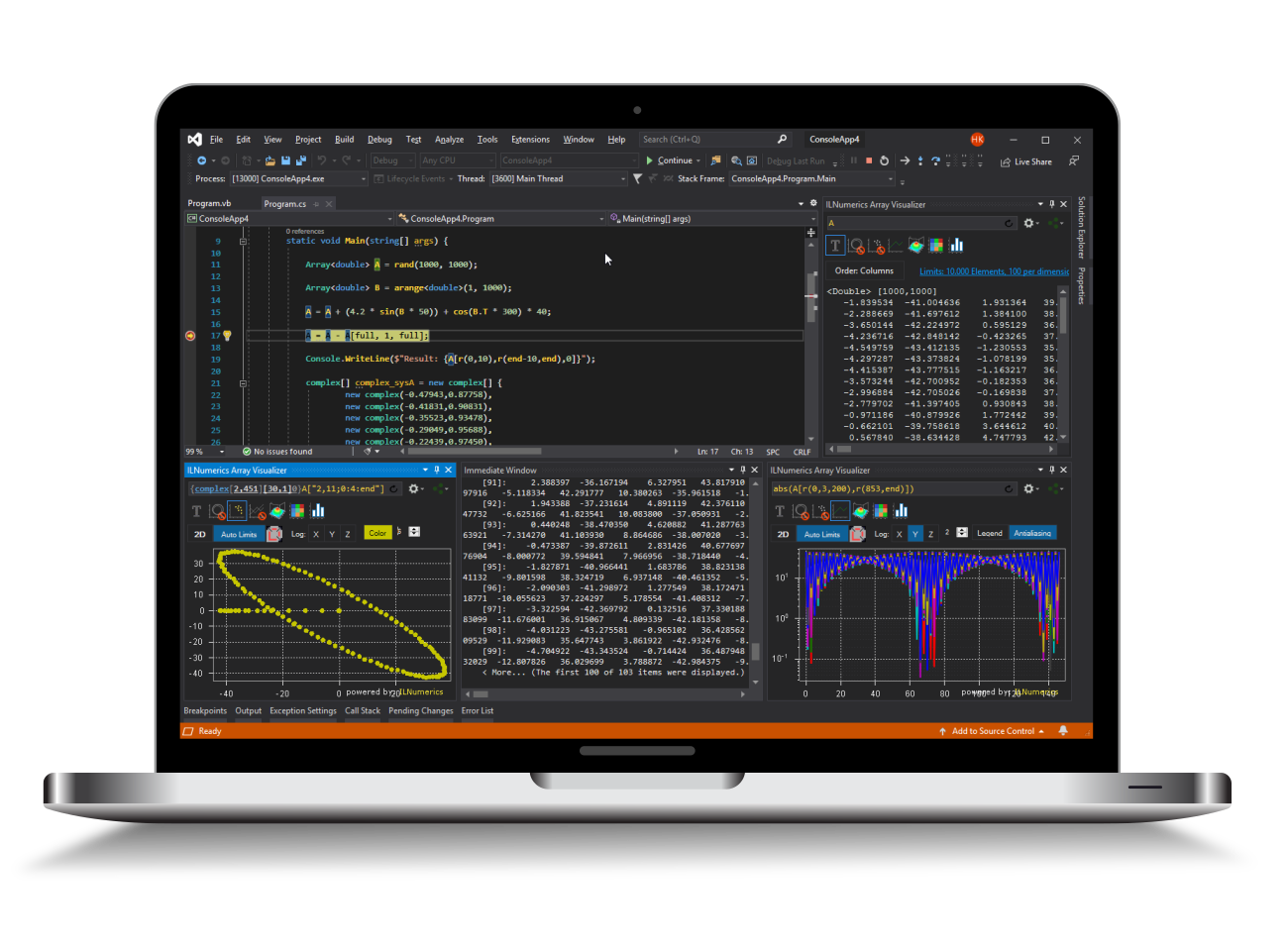Industrial Data Science
in C# and .NET:
Simple. Fast. Reliable.ILNumerics - Technical Computing

Modern High Performance Tools for Technical

Computing and Visualization in Industry and Science

tgt

# Linear Least Squares Methods in .NET (C# and Visual Basic)

Linear least squares fit, or ordinary least squares fitting methods are very popular in the context of data fitting in statistics, economics, and engineering, to name only a few. The goal is to fit a model estimator to actual data in a least squares sense, i.e. minimizing the sum of squared differences between the observed data and the values produced by the model. The 'better' the model matches the unknown underlying process which generated the values, the smaller the differences (errors or residuals) will be.

Linear least squares problems assume a linearity in the estimator parameters: a linear dependence exists between the model parameters and the outcoming errors. This is the major distinction between ordinary least squares and nonlinear least squares methods. While latter, in general, have a non-closed form and are solved via iterative methods, linear least squares on the other hand have a closed form and a unique solution which is guaranteed to be found in a limited number of computational steps.

Here are examples for a linear regression and a polynomial ridge regression.